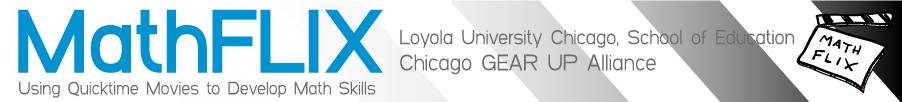### Content Index: MMagic Square - Multiplication

• Number & Operations
• Multiples
• Multiplication
• alternative algorithms (methods)
• computer integration
• distance formulas
• exponents
• factorials
• fractions
• geometric sequence
• Happy numbers
• multiples
• optical illusion
• order of operations
• permutations
• proportional reasoning
• puzzles
• relationship to surface area
• strategies for mastery of facts
• surface area
• using logarithms
• using multiples of 10, 100, 1000
• volume
• volume (see: cube numbers)